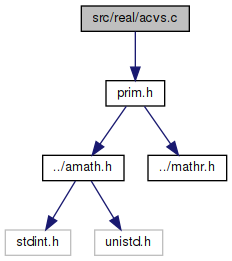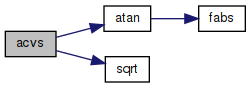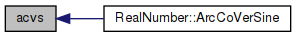amath  1.8.5 Simple command line calculator
acvs.c File Reference

Inverse coversed sine function. More...

`#include "prim.h"`
Include dependency graph for acvs.c:Go to the source code of this file.

## Functions

double acvs (double x)
Inverse coversed sine function. More...

## Detailed Description

Inverse coversed sine function.

Definition in file acvs.c.

## ◆ acvs()

 double acvs ( double x )

Inverse coversed sine function.

Definition at line 40 of file acvs.c.

References atan(), and sqrt().

Referenced by RealNumber::ArcCoVerSine().

41 {
42  double a = 1.0 - x;
43  double b = 2.0 * x - x * x;
44  double c = sqrt(b);
45  double d = atan(a / c);
46  return d;
47 }
double atan(double x)
Inverse tangent function.
Definition: atan.c:103
double sqrt(double x)
Square root function.
Definition: sqrt.c:119
Here is the call graph for this function:Here is the caller graph for this function: